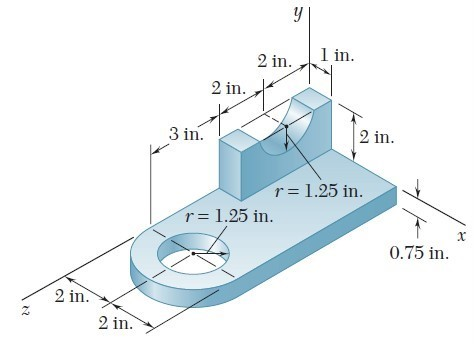# CIVE 260 Homework 5

## Problem 1

Determine the horizontal and vertical components of force at pins A, B, and C, and the reactions at the fixed support D of the three-member frame.

(Ans. FAC = 8.33 kN)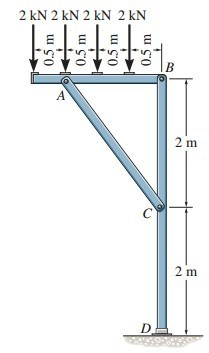## Problem 2

Determine the force P needed to support the 20-kg mass using the Spanish Burton rig. Also, what are the reactions at the supporting hooks A, B, and C?

(Ans. RA = 43.6 N)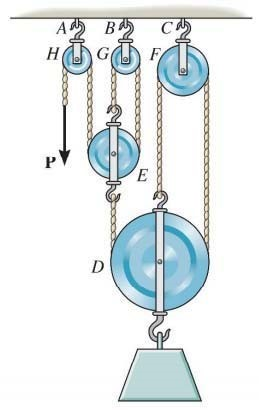## Problem 3

Determine the force exerted on the small-diameter bar (AC). (Ans. FAC = 8.52 kN)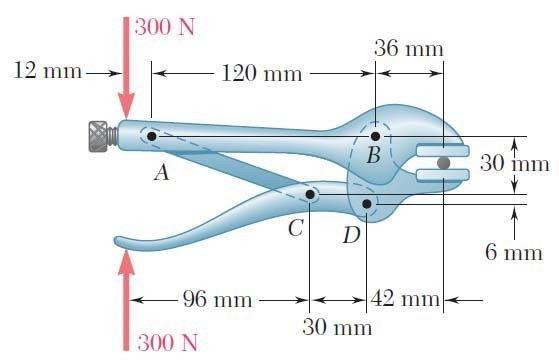## Problem 4

The loader carries the load shown. The bucket’s motion is controlled by two identical mechanisms (only one is shown) that together support the load. Determine:

• The force in cylinder CD
• The force in cylinder FH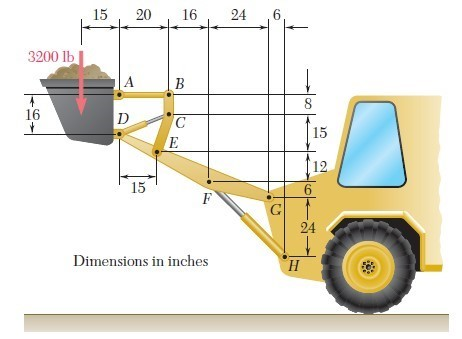## Problem 5

Replace the loading with an equivalent resultant force, and specify its location on the beam measured from point B.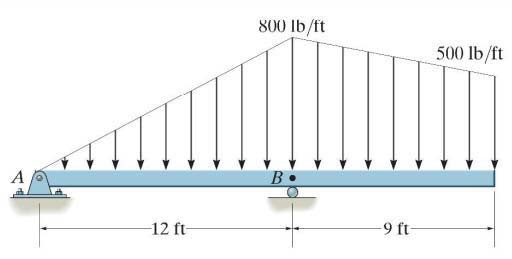## Problem 6

Determine the support reactions on the beam.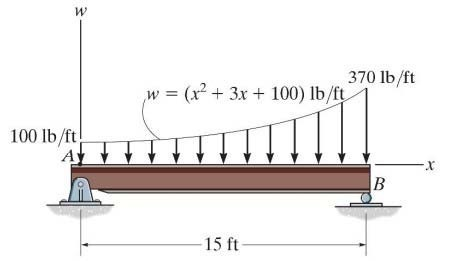## Problem 7

Determine the location of center of mass in the following steel plate.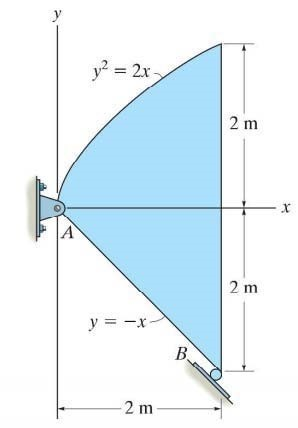## Problem 8

For the machine element shown, locate the z coordinate of the center of gravity.